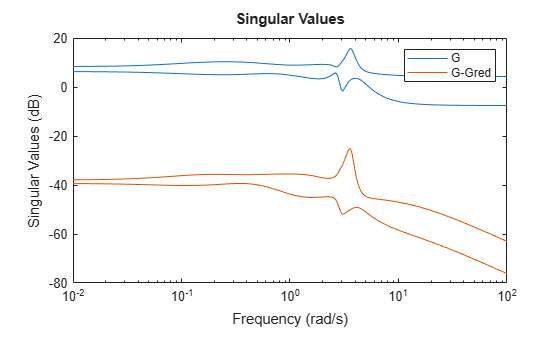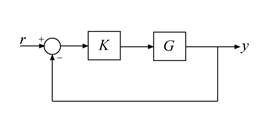# ncfmr

Model reduction from normalized coprime factorization

## Syntax

``[Gred,info] = ncfmr(G,ord)``
``[~,info] = ncfmr(G)``
``Gred = ncfmr(G,ord,info)``
``ncfmr(G)``

## Description

`ncfmr` computes a reduced-order approximation of a model by truncating modes in a coprime factorization of the full-order model. This method is related to the balanced truncation method of `balred`, but it is particularly well-suited to controller order reduction. For a stabilizing controller, the reduced controller is also stabilizing as long as the approximation error is smaller than the robustness margin computed by `ncfmargin`.

example

````[Gred,info] = ncfmr(G,ord)` computes a reduced-order approximation of the dynamic system model `G`. Specify the desired reduction order as `ord`. If `ord` is a vector, then `Gred` is an array of approximations of the corresponding order. The structure `info` contains information about the computation such as bounds on the approximation error.```

example

````[~,info] = ncfmr(G)` computes the coprime factorization of `G` given by `[M,N]` such that `G = M\N` (see `lncf`), the Hankel singular values of the factorization, and the error bounds. You can use this information to determine the target reduction order programmatically based on desired fidelity or robust stability considerations. Then, use the syntax ```Gred = ncfmr(G,ord,info)``` to compute the reduced-order model.```

example

````Gred = ncfmr(G,ord,info)` computes the reduced-order approximation using the normalized coprime factorization and Hankel singular values that you provide in `info`. Obtain `info` using the previous syntax, ```[~,info] = ncfmr(G)```. Providing a previously computed `info` to `ncfmr` allows you to perform model reduction without having to recompute the factorization and Hankel singular values. This syntax is therefore particularly useful when performance is a concern. ```

example

````ncfmr(G)` plots the Hankel singular values and bounds on the approximation error corresponding to each order. Examine the plot to determine a reduced order based on desired fidelity or robust stability considerations. You can then use `Gred = ncfmr(G,ord)` to compute the reduced-order model.```

## Examples

collapse all

`ncfmr` computes Hankel singular values and approximation errors to help you select a suitable target reduction order. One way to do so is to examine a plot of these values. Load the 30-state plant model `G`.

```load("ncfmrModel.mat","G") size(G)```
```State-space model with 2 outputs, 3 inputs, and 30 states. ```

Call `ncfmr` without an output argument. The function generates a Hankel singular value plot, which shows the relative energy contributions of each state in the coprime factorization of `G`, arranged in decreasing order by energy. The plot also shows the upper bound on the error between the original and reduced-order models that you obtain by truncating the states at that point. Examine this plot to choose the target order. For instance, for a maximum error of 0.01, you can reduce the model to 13th order.

`ncfmr(G)`Call `ncfmr` again with an output argument and using `order` = 13. Doing so computes the reduced model `Gred`. Examine the singular values of `G` and of the difference between `G` and `Gred`. The difference is very small across all frequencies, showing that the reduced-order model is a good approximation of the full-order model.

```Gred = ncfmr(G,13); sigma(G,G-Gred) legend("G","G-Gred")```When you use `ncfmr` to reduce a plant `G` or controller `K` for which the closed-loop response `feedback(G*K,eye(n))` is stable, the resulting closed-loop response is also stable as long as the approximation error of the reduced model does not exceed the robustness margin computed by `ncfmargin`. To see this benefit of `ncfmr`, load a plant `G` and design a controller for it. For this example, use `ncfsyn` to design the controller.

```load ncfmrStability.mat G size(G)```
```State-space model with 1 outputs, 1 inputs, and 3 states. ```
```% shaping weights s = tf('s'); W1 = 3.35*tf([1 20.89],[1 0]); W2 = 1; % controller [K,~,~,Kinfo] = ncfsyn(G,W1,W2); size(K)```
```State-space model with 1 outputs, 1 inputs, and 5 states. ```

`ncfsyn` designs a controller by optimizing the `ncfmargin` robustness margin using a plant shaped by weighting functions `W1` and `W2` (see `ncfsyn`). To analyze margins with `ncfmargin` and reduce controller order with `ncfmr`, work with the shaped plant `Gs` and the controller `Ks` designed for it.

```Gs = Kinfo.Gs; Ks = Kinfo.Ks;```

Use `ncfmargin` to find the robustness margin of the system with the full-order controller. `ncfsyn` assumes a positive feedback loop while `ncfmargin` assumes negative feedback, so reverse the sign of the controller for this computation.

`emax = ncfmargin(Gs,-Ks)`
```emax = 0.1956 ```

As long as the approximation error of the reduced-order controller does not exceed `emax`, stability of the closed-loop system is preserved. Suppose that you can tolerate up to a 50% reduction in this margin in exchange for the computational benefit of a lower order controller. To select the reduced order, first compute the errors associated with each target order. `ncfmr` returns these values in the `ErrorBound` field of the `info` argument. Then find the index of the last entry in `info.ErrorBound` that exceeds the target error of `emax/2`.

```[~,info] = ncfmr(Ks); r = find(info.ErrorBound>emax/2,1,'last')```
```r = 3 ```

Thus, you can approximate the original controller by only three states without too much loss of stability. To avoid recomputing the Hankel singular values of `Ks`, use `info` as an input argument to `ncfmr`.

```Ksr = ncfmr(Ks,r,info); size(Ksr)```
```State-space model with 1 outputs, 1 inputs, and 3 states. ```

The reduced-order controller yields a very similar stability margin to the original controller.

`ncfmargin(Gs,-Ksr)`
```ans = 0.1949 ```

Reducing the controller order further leads to additional reduction in the stability margin. Reducing too far can lead to loss of closed-loop stability. For instance, try reducing to first order.

```Ksru = ncfmr(Ks,1,info); ncfmargin(Gs,-Ksru)```
```ans = 0 ```

Thus, for further analysis or implementation, use the third-order controller. To do so, convert `Ksr`, the reduced controller for `Gs`, into `Kr`, the reduced controller for `G`.

`Kr = W1*Ksr*W2;`

To confirm that this controller is satisfactory, compare the closed-loop response to the response with the full-order controller. Again, reverse the sign of the controller to account for `ncfsyn` assuming positive feedback.

```CL = feedback(-G*K,1); CLr = feedback(-G*Kr,1); step(CL,CLr) legend```The large overshoot in this case is due to instability of the original plant `G`.

`ncfmr` can compute multiple reduced-order models at once and return them in a model array. This can be useful, for example, when you want to test a controller design with multiple approximations to choose the one that yields the best balance between accuracy and computational efficiency. To compute multiple models, provide a vector of target reduction orders instead of a single value for `order`.

Load the 30-state plant model `G`. Compute five approximations of orders 11−15.

```load("ncfmrModel.mat","G") orders = 11:15; Gred = ncfmr(G,orders); size(Gred)```
```5x1 array of state-space models. Each model has 2 outputs, 3 inputs, and between 11 and 15 states. ```

`Gred` is an array of reduced-order state-space (`ss`) models. You can use the `SamplingGrid` property of `ss` to associate each entry in the array with its corresponding model order.

`Gred.SamplingGrid = struct('order',orders);`

Assigning `SamplingGrid` can be useful for keeping track of the entries in a model array. For instance, if you plot the frequency response of `Gred` in a MATLAB® figure, clicking one of the resulting responses creates a tooltip that includes information drawn from `SamplingGrid`.

## Input Arguments

collapse all

Model to reduce, specified as a dynamic system model such as a state-space (`ss`) model. `G` can be stable or unstable. If `G` is a generalized state-space model with uncertain or tunable control design blocks, then the function uses the nominal or current value of those elements. `sys` cannot be an `frd` model or a model with time delays.

Reduction order, specified as a positive integer or a vector of positive integers. If `ord` is a scalar, `ncfmr` returns the model `Gred` of that order. If `ord` is a vector, then `Gred` is an array of models reduced to the corresponding orders.

To determine `ord`, you can use one of two methods:

• Use the syntax `ncfmr(G)` to obtain a plot of Hankel singular values and bounds on approximation errors at each order. Examine the plot to choose a reduction order with a tolerable approximation error. For an example, see Reduce Model Order.

• Use the syntax `[~,info] = ncfmr(G)` to obtain the `info` structure. Programmatically examine the approximation error bounds in `info.ErrorBounds` to choose a reduction order. For an example, see Reduce Controller Order While Preserving Stability and Robustness.

If `G` has unstable states, then `ord` must be at least the number of unstable states.

## Output Arguments

collapse all

Reduced-order model, returned as a state-space (`ss`) model. If `ord` is a scalar, then `Gred` is a single model of order `ord`. If `ord` is a vector, then `Gred` is an array of `ss` models of corresponding orders.

Information about model-reduction calculation, returned as a structure with the following fields.

• `GL` — Left normalized coprime factorization of `G`, returned as a state-space (`ss`) model. This factorization is given by `GL = lncf(G)`. For more information, see `lncf`.

• `HSV` — Hankel singular values of `GL`, returned as a vector whose length is the number of states in `G`. These values indicate the relative energy contribution of each state. You can choose a target reduction order by examining these values and choosing a number of states after which the energy contribution drops off significantly.

• `ErrorBound` — Upper bound on approximation errors, returned as a vector. The approximation error is given by ${‖\left[{M}_{r},{N}_{r}\right]-\left[M,N\right]‖}_{\infty }$, where [M,N] = `lncf(G)` and [Mr,Nr] = `lncf(Gred)`. (For more information about these expressions, see `lncf`.) Each entry `info.ErrorBound(j)` is the maximum approximation error associated with reducing to `j` states. Thus, for instance, if you want an approximation error of no more than 0.01, examine `info.ErrorBound` to find the index of the first entry that is less than 0.01. Use that index as `ord`.

## Tips

• You can use `ncfmr` to reduce the plant G or controller K while preserving closed-loop stability of the following SISO or MIMO feedback loop.Stability of this loop is preserved as long as the approximation error of the reduced plant is smaller than the robustness margin for this loop given by `ncfmargin(G,K)`.

For controllers computed with `ncfsyn`, reducing the controller Ks that `ncfsyn` computes for the shaped controller Gs is preferable. Both Ks and Gs are returned by `ncfsyn` in the `info` output argument. You can then compute Kr, the reduced controller for the original plant G, from Kr = W1KsrW2, where W1 and W2 are the shaping weights used with `ncfsyn`. For an example, see Reduce Controller Order While Preserving Stability and Robustness.

For controllers obtained by other techniques, reduction with `ncfmr` also preserves stability if the error does not exceed the `ncfmargin` margin. However, such reduction can partially remove integral action and introduce steady-state tracking errors. Therefore, removing any integrator terms from the controller before reduction with `ncfmr` and replacing them in the reduced controller is recommended.

## Algorithms

`ncfmr` performs the following steps to reduce the input model G to the desired order k.

1. Find the left normalized coprime factorization [M,N] of `G`, where `G` = M\N (see `lncf`).

2. Obtain the kth-order approximation [Mr,Nr] of [M,N], using balanced model truncation with absolute error control (see `balred`).

3. Set `Gred` = Mr\Nr.

## Version History

Introduced before R2006a

expand all